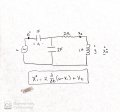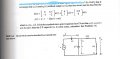# Need clarification on equation used to model part of an RLC circuit

#### seand33ly

Joined Sep 13, 2019
1The equation above is used to describe part of the circuit above. I am looking for help understanding where this equation came from. I was able to derive equations for x2' and y successfully but I cannot figure out how x1' is derived.

Below is the original problem.

Please let me know if you have any questions/need further clarification. Any help is appreciated! Thank you.